Скачать презентацию CHAPTER 8 Stocks and Their Valuation n n

f5fdaa5f2ed9547dfd21e904637fd1b9.ppt

• Количество слайдов: 39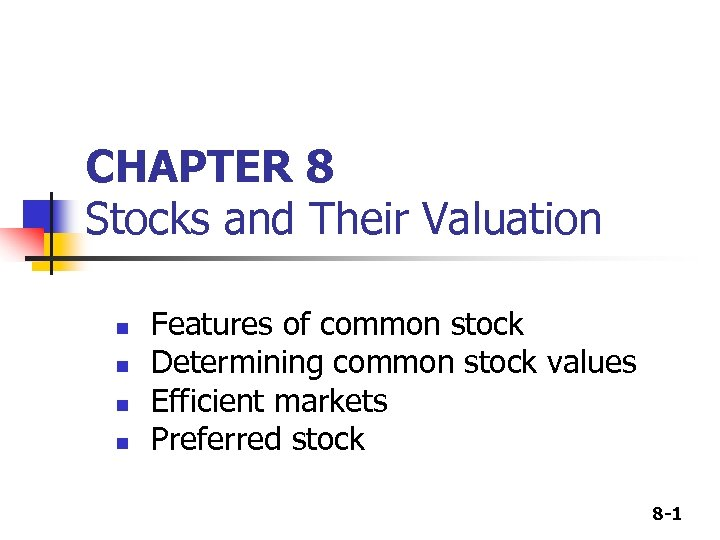CHAPTER 8 Stocks and Their Valuation n n Features of common stock Determining common stock values Efficient markets Preferred stock 8 -1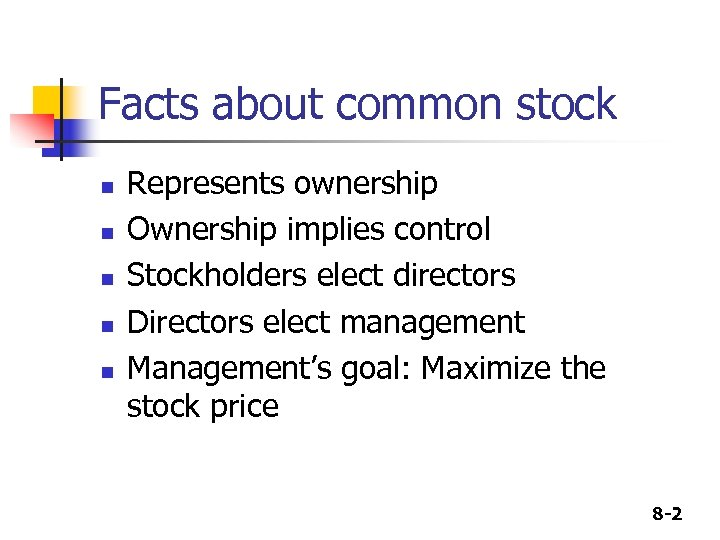Facts about common stock n n n Represents ownership Ownership implies control Stockholders elect directors Directors elect management Management’s goal: Maximize the stock price 8 -2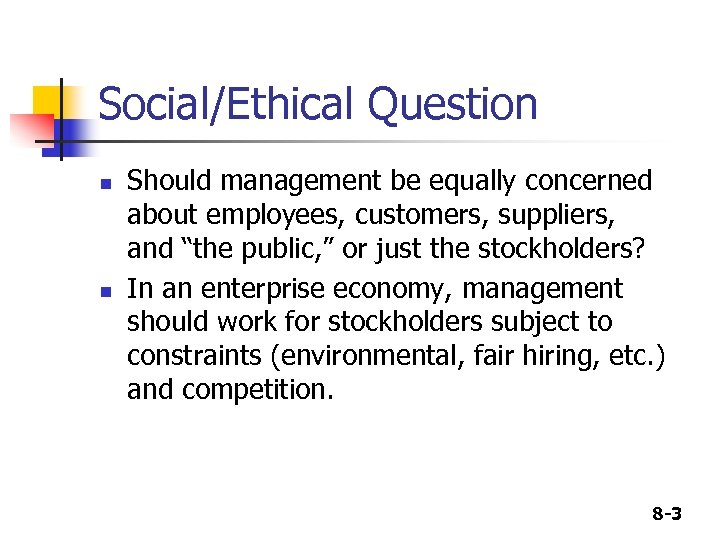Social/Ethical Question n n Should management be equally concerned about employees, customers, suppliers, and “the public, ” or just the stockholders? In an enterprise economy, management should work for stockholders subject to constraints (environmental, fair hiring, etc. ) and competition. 8 -3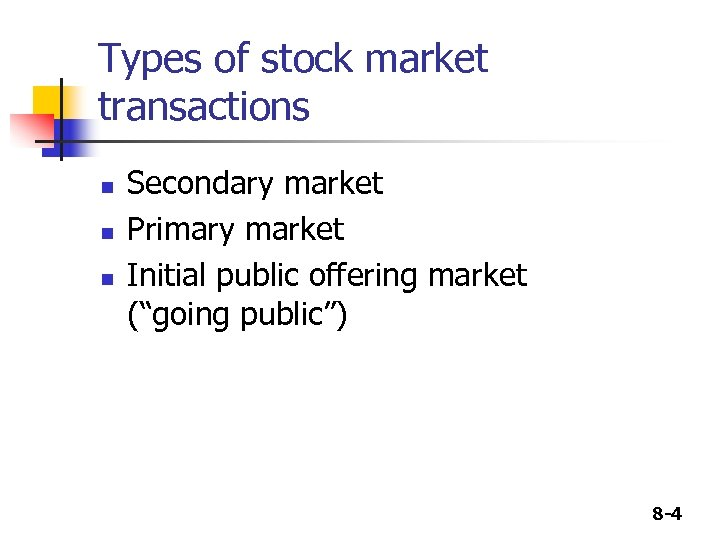Types of stock market transactions n n n Secondary market Primary market Initial public offering market (“going public”) 8 -4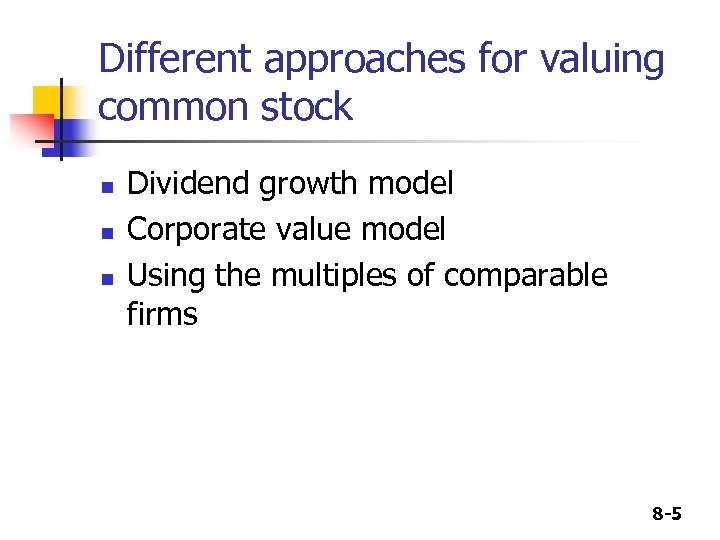Different approaches for valuing common stock n n n Dividend growth model Corporate value model Using the multiples of comparable firms 8 -5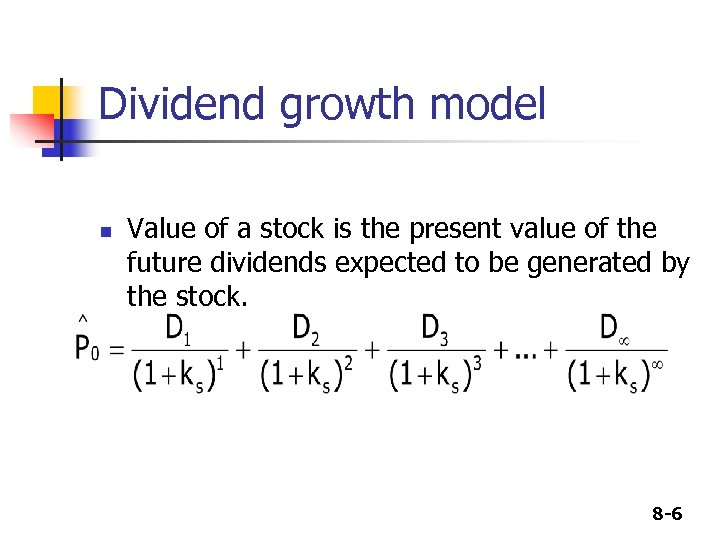Dividend growth model n Value of a stock is the present value of the future dividends expected to be generated by the stock. 8 -6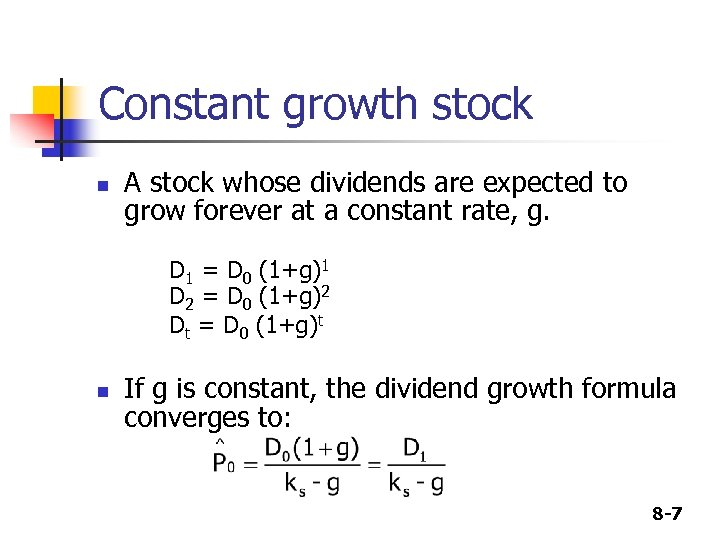Constant growth stock n A stock whose dividends are expected to grow forever at a constant rate, g. D 1 = D 0 (1+g)1 D 2 = D 0 (1+g)2 Dt = D 0 (1+g)t n If g is constant, the dividend growth formula converges to: 8 -7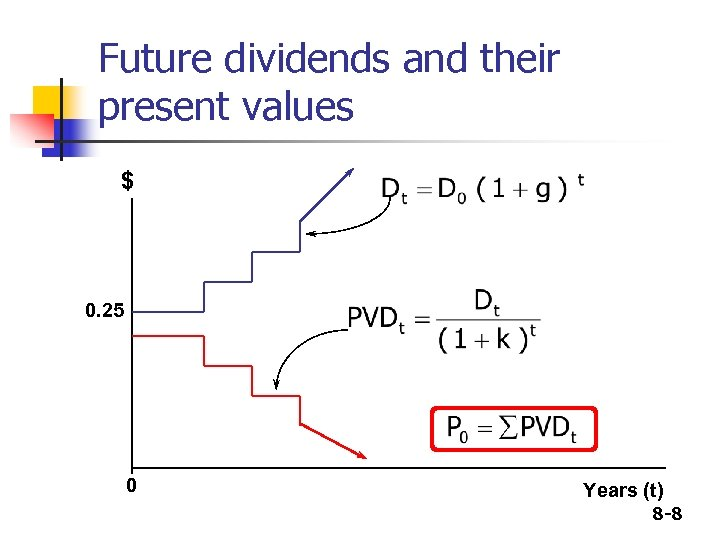Future dividends and their present values \$ 0. 25 0 Years (t) 8 -8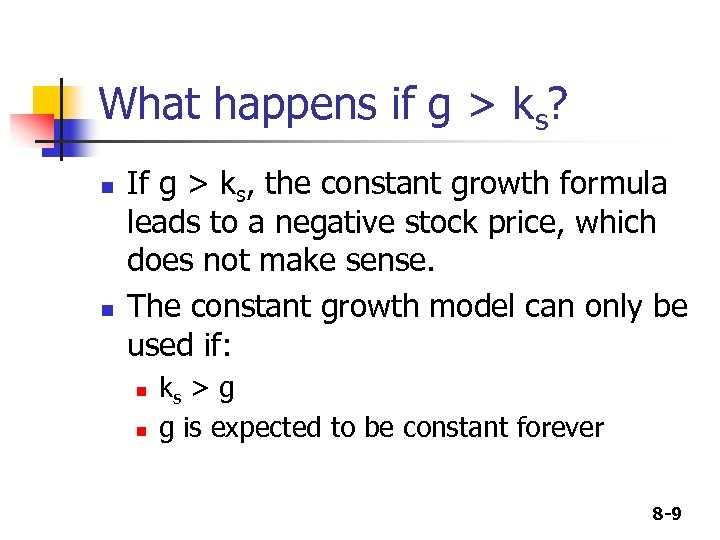What happens if g > ks? n n If g > ks, the constant growth formula leads to a negative stock price, which does not make sense. The constant growth model can only be used if: n n ks > g g is expected to be constant forever 8 -9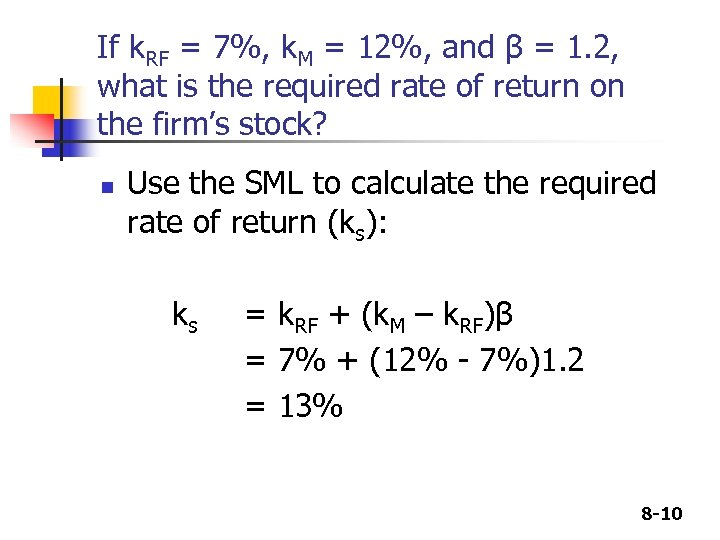If k. RF = 7%, k. M = 12%, and β = 1. 2, what is the required rate of return on the firm’s stock? n Use the SML to calculate the required rate of return (ks): ks = k. RF + (k. M – k. RF)β = 7% + (12% - 7%)1. 2 = 13% 8 -10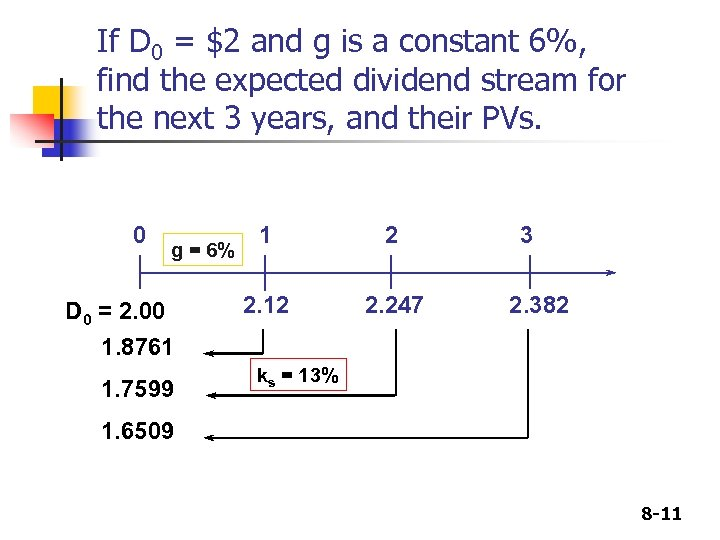If D 0 = \$2 and g is a constant 6%, find the expected dividend stream for the next 3 years, and their PVs. 0 g = 6% D 0 = 2. 00 1 2 2. 12 2. 247 3 2. 382 1. 8761 1. 7599 ks = 13% 1. 6509 8 -11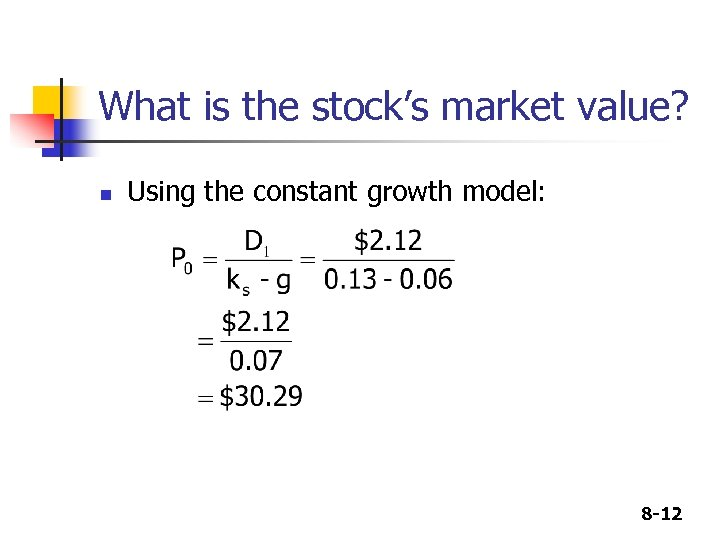What is the stock’s market value? n Using the constant growth model: 8 -12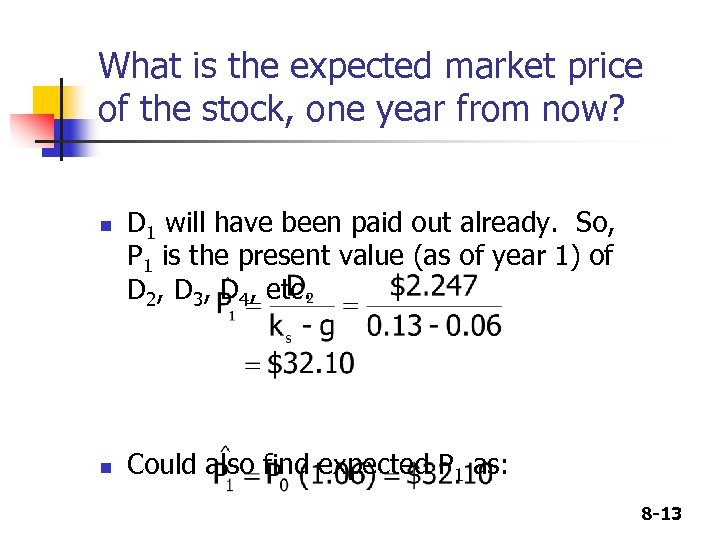What is the expected market price of the stock, one year from now? n n D 1 will have been paid out already. So, P 1 is the present value (as of year 1) of D 2, D 3, D 4, etc. Could also find expected P 1 as: 8 -13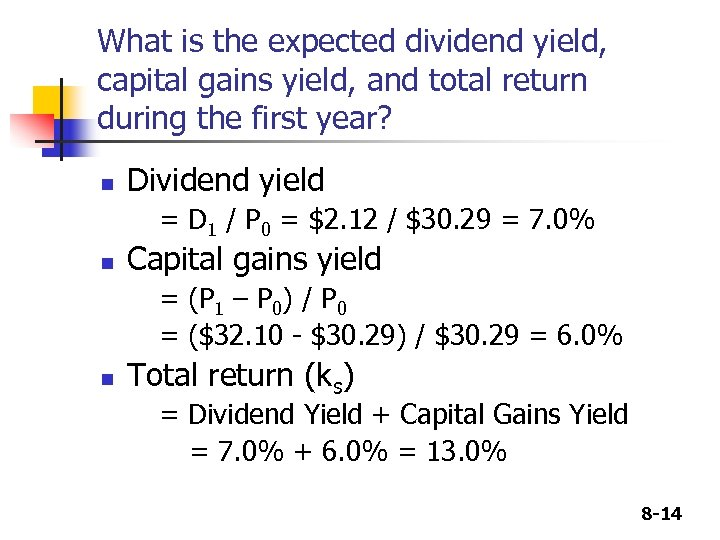What is the expected dividend yield, capital gains yield, and total return during the first year? n Dividend yield = D 1 / P 0 = \$2. 12 / \$30. 29 = 7. 0% n Capital gains yield = (P 1 – P 0) / P 0 = (\$32. 10 - \$30. 29) / \$30. 29 = 6. 0% n Total return (ks) = Dividend Yield + Capital Gains Yield = 7. 0% + 6. 0% = 13. 0% 8 -14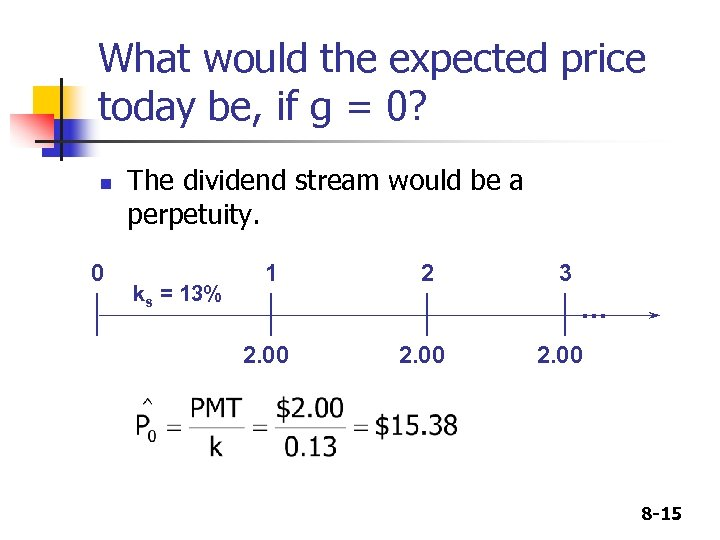What would the expected price today be, if g = 0? n 0 The dividend stream would be a perpetuity. ks = 13% 1 2 3 . . . 2. 00 8 -15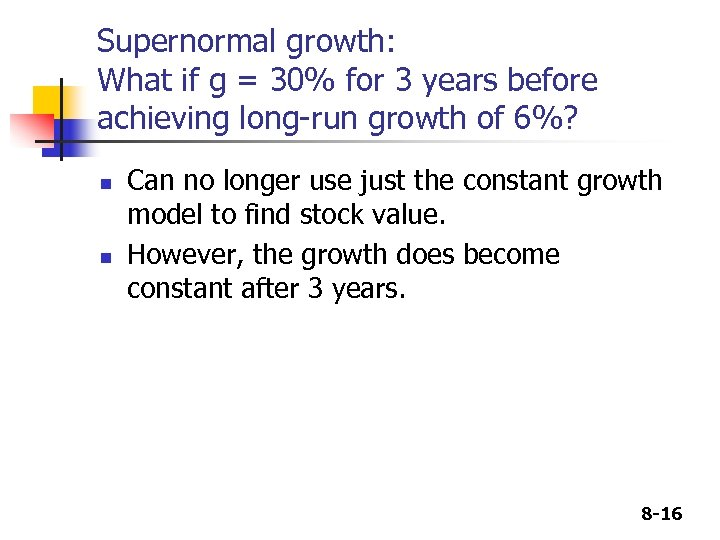Supernormal growth: What if g = 30% for 3 years before achieving long-run growth of 6%? n n Can no longer use just the constant growth model to find stock value. However, the growth does become constant after 3 years. 8 -16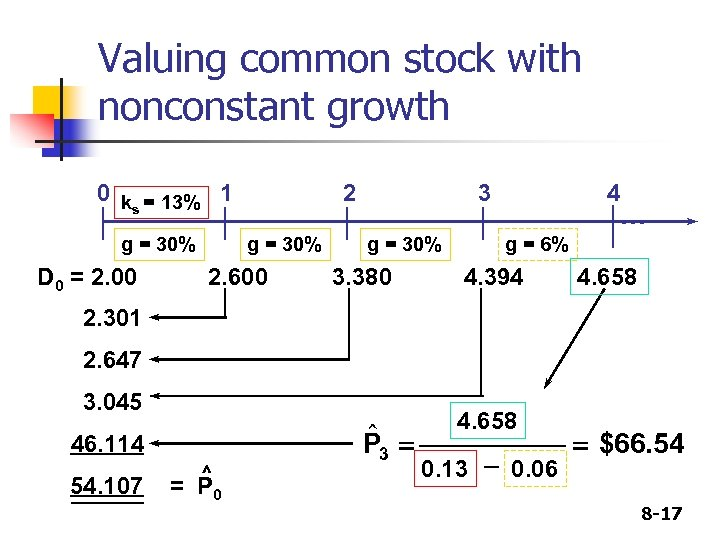Valuing common stock with nonconstant growth 0 k = 13% 1 s g = 30% D 0 = 2. 00 2 g = 30% 2. 600 3 g = 30% 3. 380 4 . . . g = 6% 4. 394 4. 658 2. 301 2. 647 3. 045 46. 114 54. 107 ^ = P 0 \$ P 3 = 4. 658 0. 13 - 0. 06 = \$66. 54 8 -17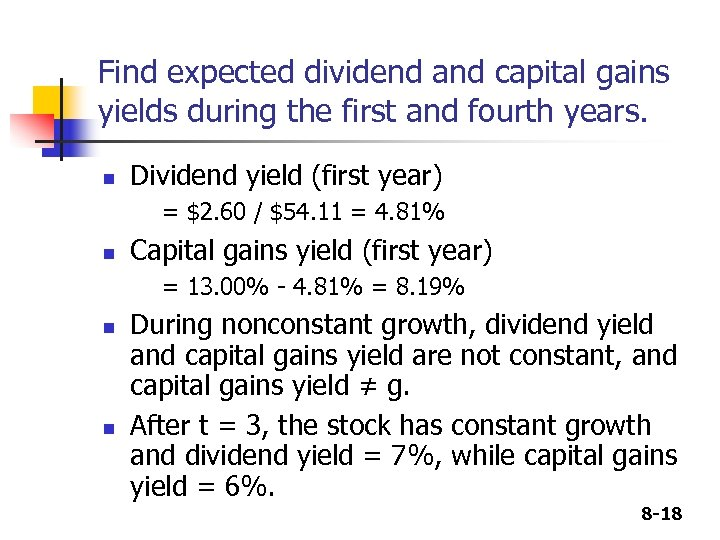Find expected dividend and capital gains yields during the first and fourth years. n Dividend yield (first year) = \$2. 60 / \$54. 11 = 4. 81% n Capital gains yield (first year) = 13. 00% - 4. 81% = 8. 19% n n During nonconstant growth, dividend yield and capital gains yield are not constant, and capital gains yield ≠ g. After t = 3, the stock has constant growth and dividend yield = 7%, while capital gains yield = 6%. 8 -18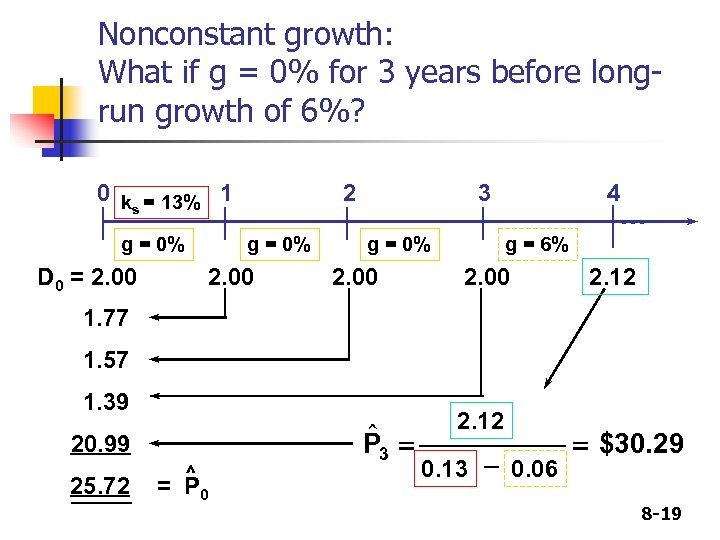Nonconstant growth: What if g = 0% for 3 years before longrun growth of 6%? 0 k = 13% 1 s g = 0% 2 g = 0% D 0 = 2. 00 3 g = 0% 2. 00 4 . . . g = 6% 2. 00 2. 12 1. 77 1. 57 1. 39 20. 99 25. 72 ^ = P 0 \$ P 3 = 2. 12 0. 13 - 0. 06 = \$30. 29 8 -19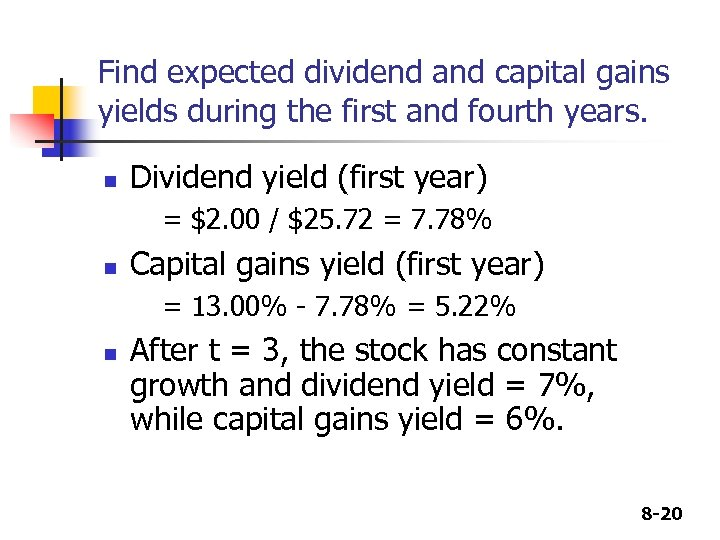Find expected dividend and capital gains yields during the first and fourth years. n Dividend yield (first year) = \$2. 00 / \$25. 72 = 7. 78% n Capital gains yield (first year) = 13. 00% - 7. 78% = 5. 22% n After t = 3, the stock has constant growth and dividend yield = 7%, while capital gains yield = 6%. 8 -20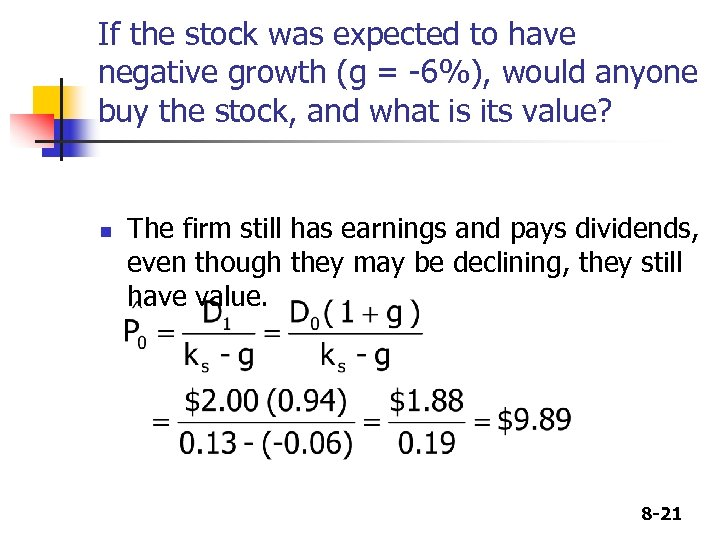If the stock was expected to have negative growth (g = -6%), would anyone buy the stock, and what is its value? n The firm still has earnings and pays dividends, even though they may be declining, they still have value. 8 -21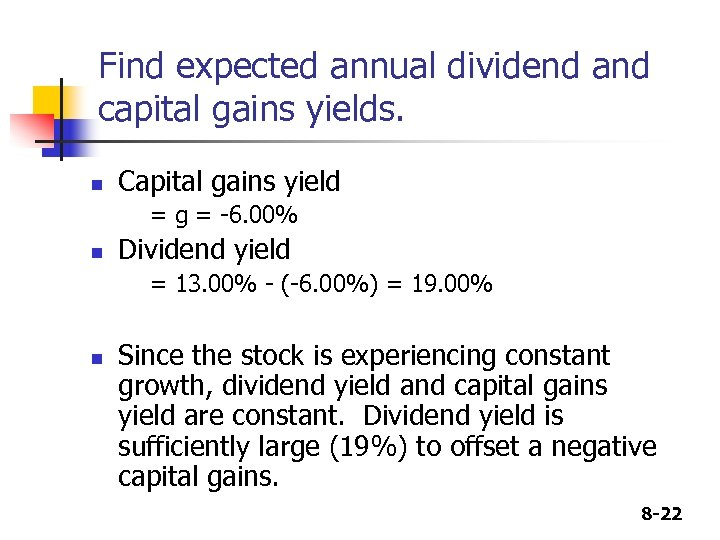Find expected annual dividend and capital gains yields. n Capital gains yield = g = -6. 00% n Dividend yield = 13. 00% - (-6. 00%) = 19. 00% n Since the stock is experiencing constant growth, dividend yield and capital gains yield are constant. Dividend yield is sufficiently large (19%) to offset a negative capital gains. 8 -22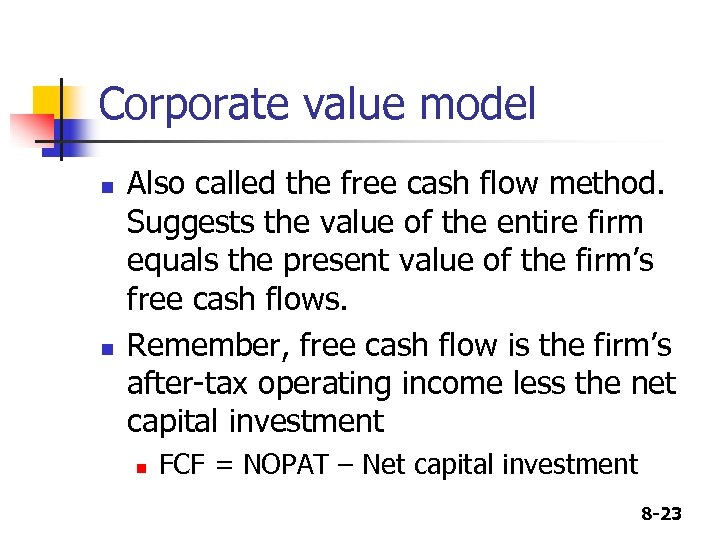Corporate value model n n Also called the free cash flow method. Suggests the value of the entire firm equals the present value of the firm’s free cash flows. Remember, free cash flow is the firm’s after-tax operating income less the net capital investment n FCF = NOPAT – Net capital investment 8 -23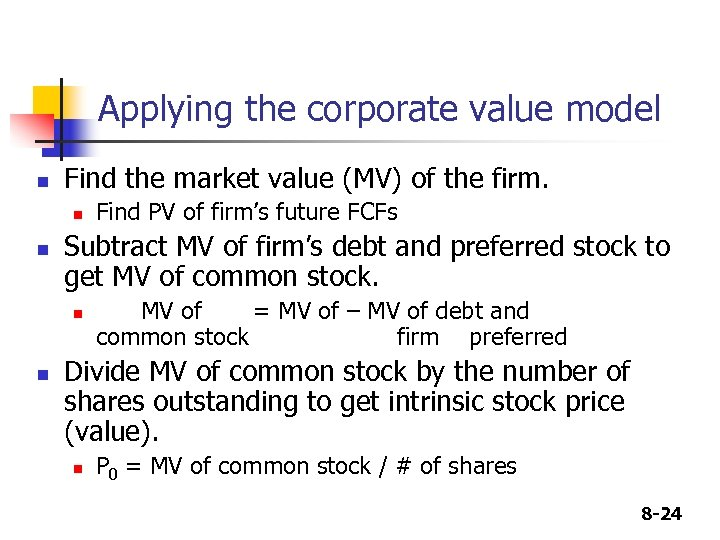Applying the corporate value model n Find the market value (MV) of the firm. n n Subtract MV of firm’s debt and preferred stock to get MV of common stock. n n Find PV of firm’s future FCFs MV of = MV of – MV of debt and common stock firm preferred Divide MV of common stock by the number of shares outstanding to get intrinsic stock price (value). n P 0 = MV of common stock / # of shares 8 -24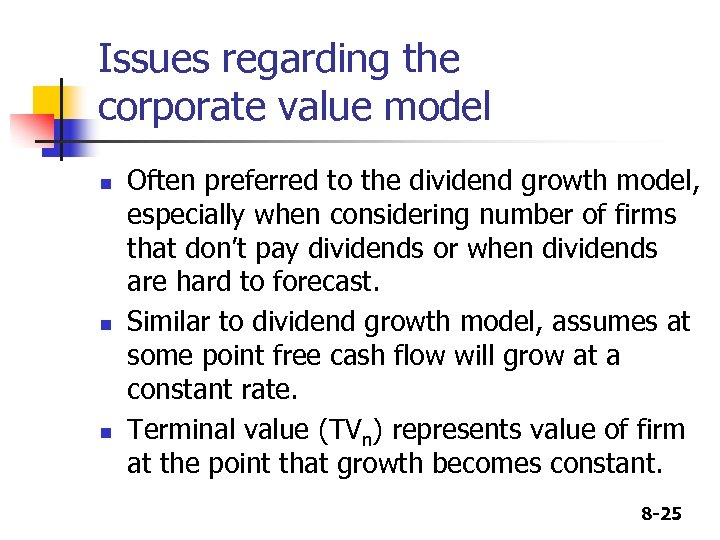Issues regarding the corporate value model n n n Often preferred to the dividend growth model, especially when considering number of firms that don’t pay dividends or when dividends are hard to forecast. Similar to dividend growth model, assumes at some point free cash flow will grow at a constant rate. Terminal value (TVn) represents value of firm at the point that growth becomes constant. 8 -25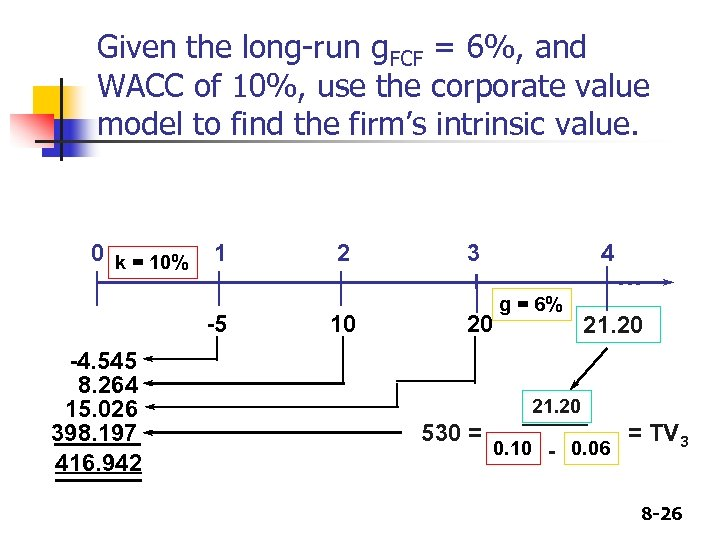Given the long-run g. FCF = 6%, and WACC of 10%, use the corporate value model to find the firm’s intrinsic value. 0 k = 10% 1 -5 -4. 545 8. 264 15. 026 398. 197 416. 942 2 10 3 4 20 g = 6% . . . 21. 20 530 = 0. 10 - 0. 06 = TV 3 8 -26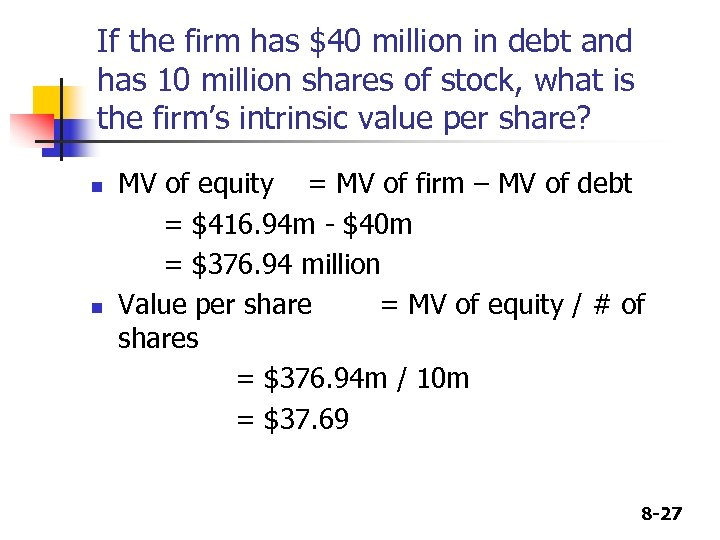If the firm has \$40 million in debt and has 10 million shares of stock, what is the firm’s intrinsic value per share? n n MV of equity = MV of firm – MV of debt = \$416. 94 m - \$40 m = \$376. 94 million Value per share = MV of equity / # of shares = \$376. 94 m / 10 m = \$37. 69 8 -27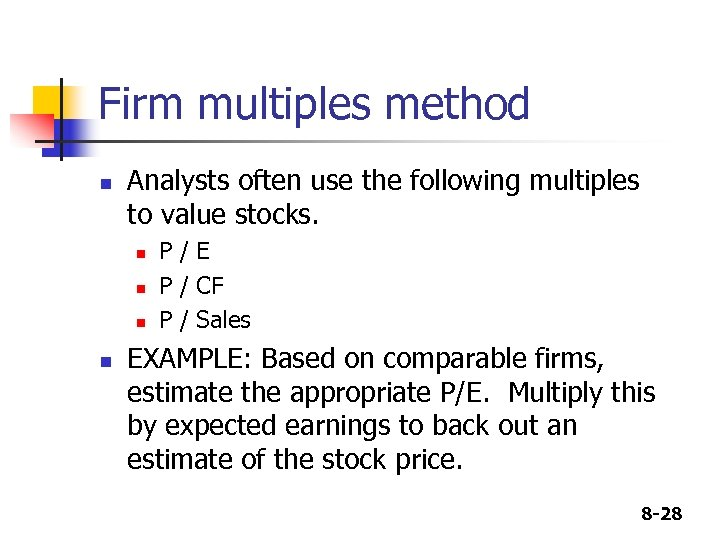Firm multiples method n Analysts often use the following multiples to value stocks. n n P/E P / CF P / Sales EXAMPLE: Based on comparable firms, estimate the appropriate P/E. Multiply this by expected earnings to back out an estimate of the stock price. 8 -28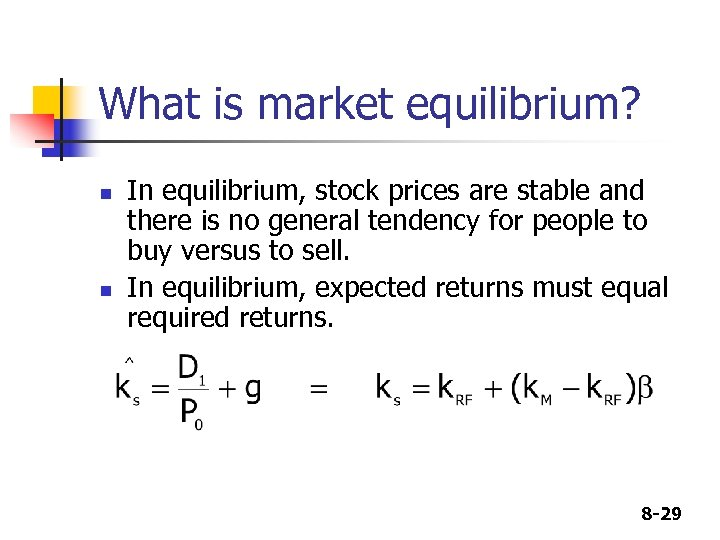What is market equilibrium? n n In equilibrium, stock prices are stable and there is no general tendency for people to buy versus to sell. In equilibrium, expected returns must equal required returns. 8 -29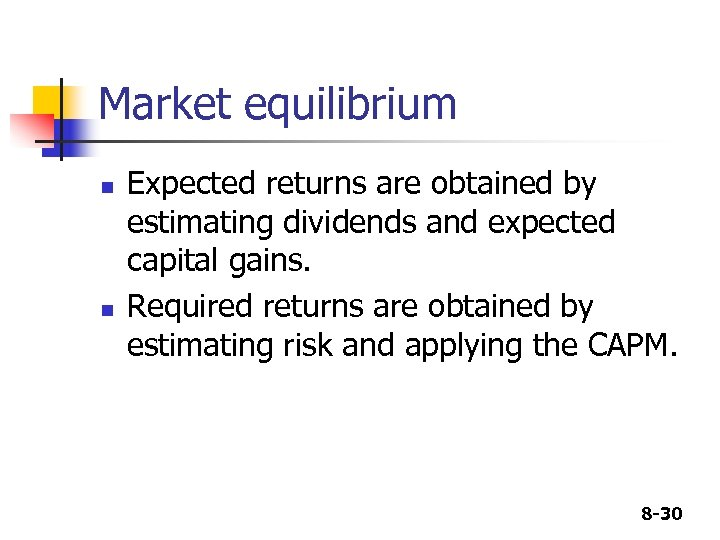Market equilibrium n n Expected returns are obtained by estimating dividends and expected capital gains. Required returns are obtained by estimating risk and applying the CAPM. 8 -30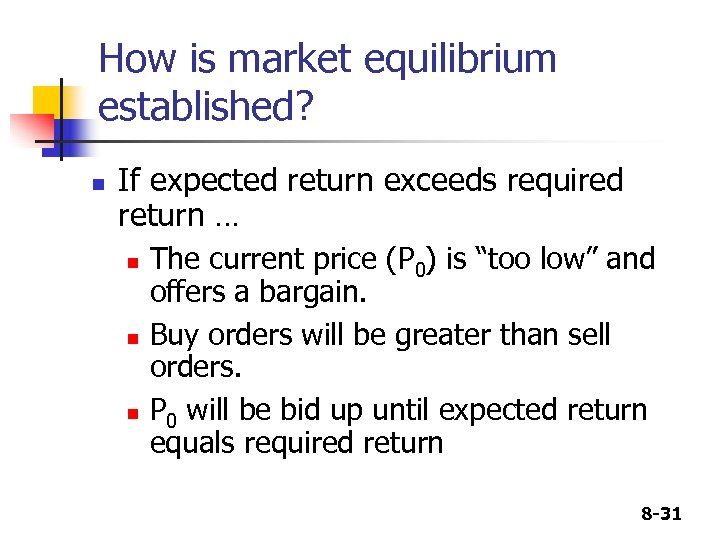How is market equilibrium established? n If expected return exceeds required return … n n n The current price (P 0) is “too low” and offers a bargain. Buy orders will be greater than sell orders. P 0 will be bid up until expected return equals required return 8 -31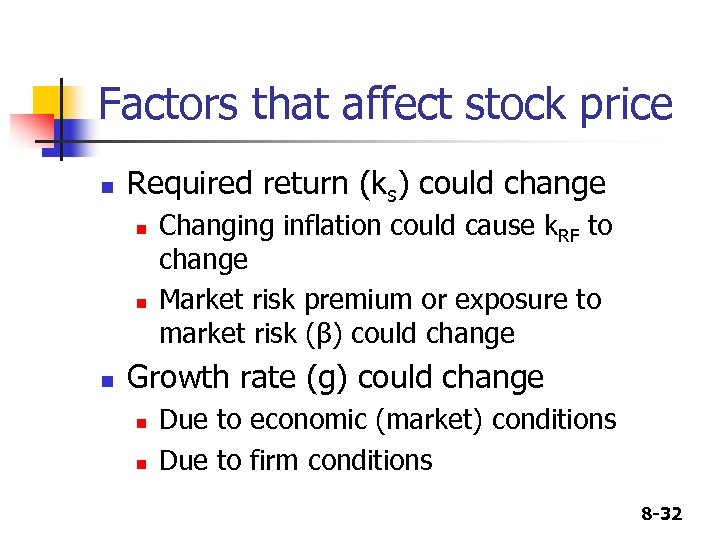Factors that affect stock price n Required return (ks) could change n n n Changing inflation could cause k. RF to change Market risk premium or exposure to market risk (β) could change Growth rate (g) could change n n Due to economic (market) conditions Due to firm conditions 8 -32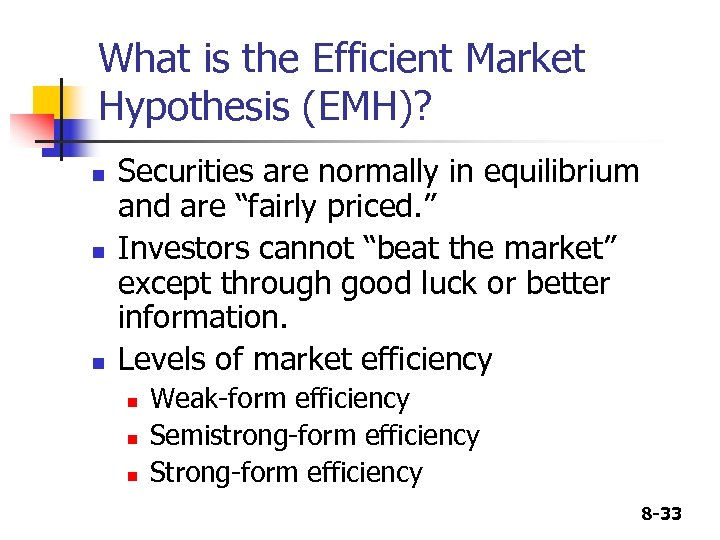What is the Efficient Market Hypothesis (EMH)? n n n Securities are normally in equilibrium and are “fairly priced. ” Investors cannot “beat the market” except through good luck or better information. Levels of market efficiency n n n Weak-form efficiency Semistrong-form efficiency Strong-form efficiency 8 -33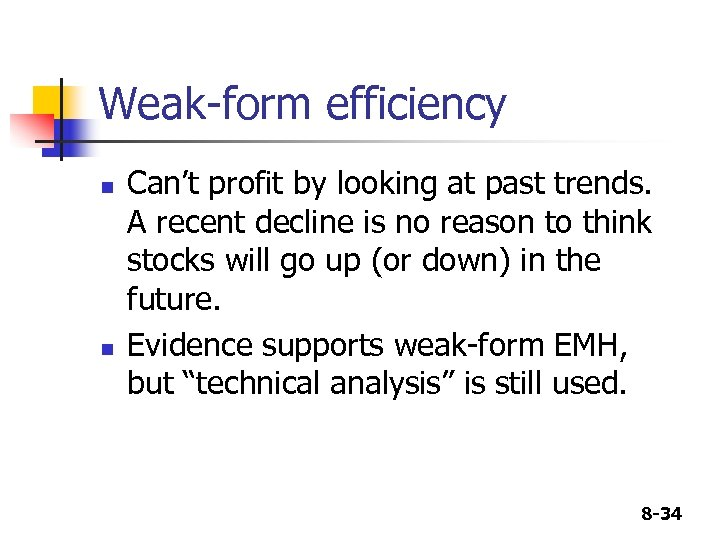Weak-form efficiency n n Can’t profit by looking at past trends. A recent decline is no reason to think stocks will go up (or down) in the future. Evidence supports weak-form EMH, but “technical analysis” is still used. 8 -34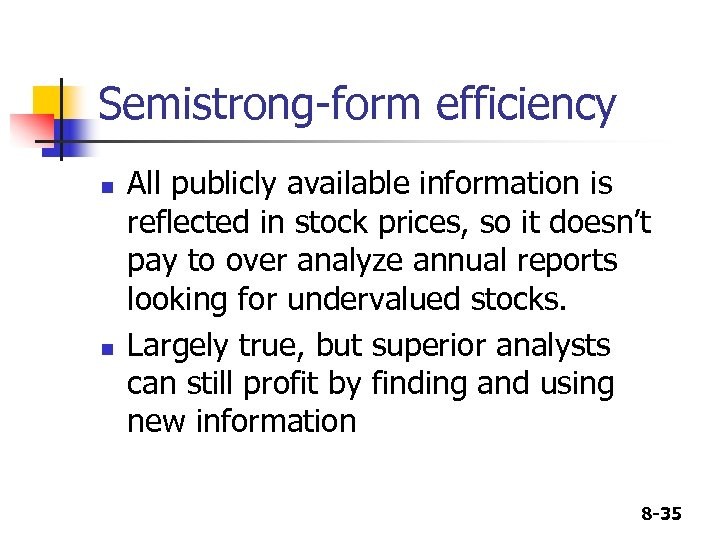Semistrong-form efficiency n n All publicly available information is reflected in stock prices, so it doesn’t pay to over analyze annual reports looking for undervalued stocks. Largely true, but superior analysts can still profit by finding and using new information 8 -35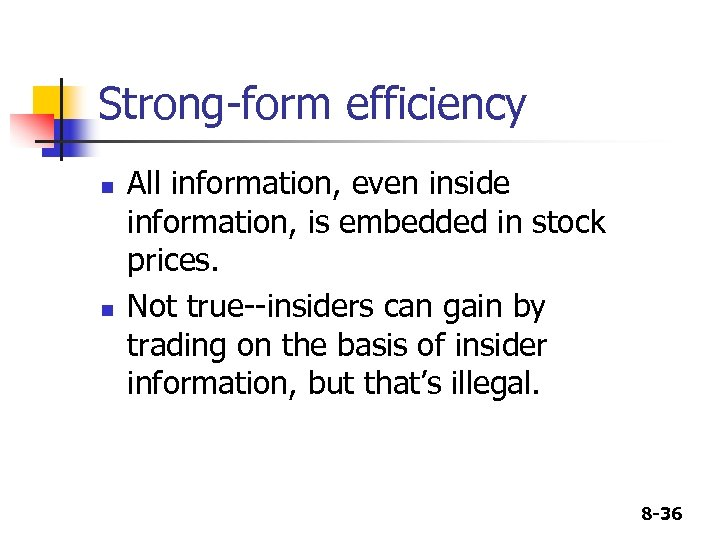Strong-form efficiency n n All information, even inside information, is embedded in stock prices. Not true--insiders can gain by trading on the basis of insider information, but that’s illegal. 8 -36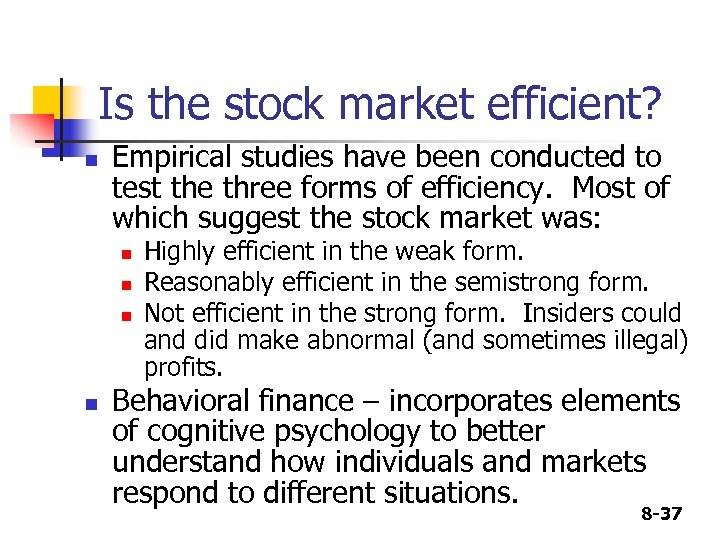Is the stock market efficient? n Empirical studies have been conducted to test the three forms of efficiency. Most of which suggest the stock market was: n n Highly efficient in the weak form. Reasonably efficient in the semistrong form. Not efficient in the strong form. Insiders could and did make abnormal (and sometimes illegal) profits. Behavioral finance – incorporates elements of cognitive psychology to better understand how individuals and markets respond to different situations. 8 -37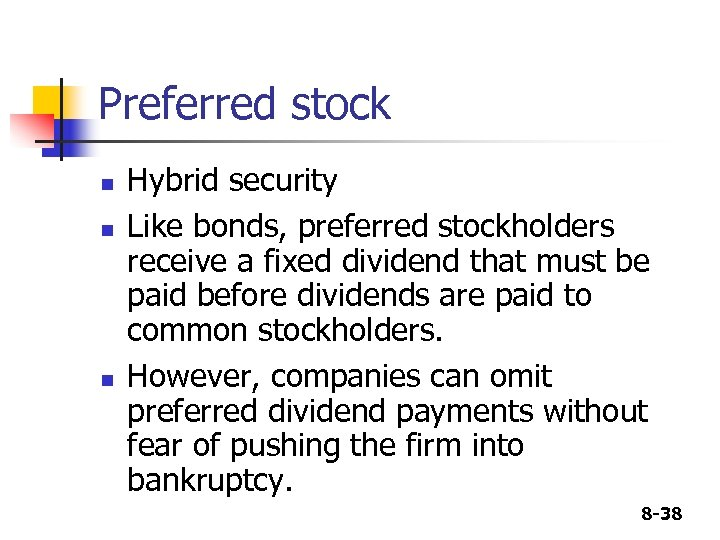Preferred stock n n n Hybrid security Like bonds, preferred stockholders receive a fixed dividend that must be paid before dividends are paid to common stockholders. However, companies can omit preferred dividend payments without fear of pushing the firm into bankruptcy. 8 -38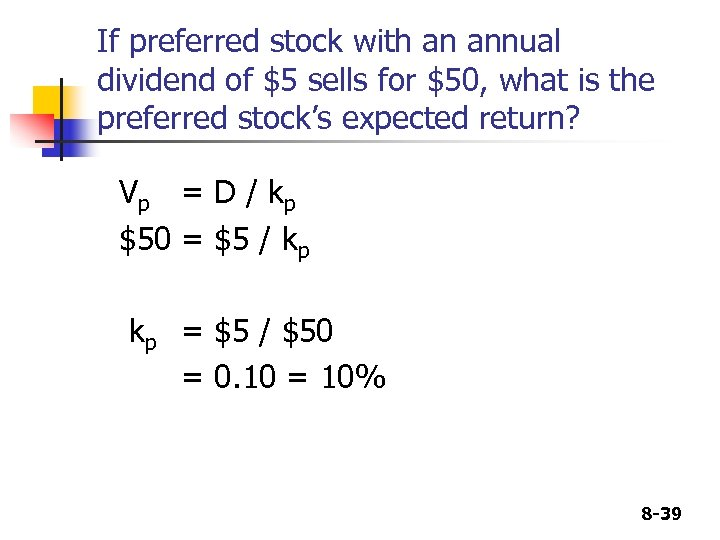If preferred stock with an annual dividend of \$5 sells for \$50, what is the preferred stock’s expected return? V p = D / kp \$50 = \$5 / kp kp = \$5 / \$50 = 0. 10 = 10% 8 -39